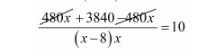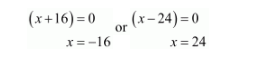# Some students planned a picnic.

Question:

Some students planned a picnic. The budget for food was Rs. 480. But eight of these failed to go and thus the cost of food for each member increased by Rs. 10. How many students attended the picnic?

Solution:

Let students planned a picnic.

Then, the share of each student $=\frac{480}{x}$

According to question, 8 students fail to go picnic, then remaining students $=(x-8)$.

Therefore, new share of each student $=\frac{480}{x-8}$

It is given that

$\frac{480}{x-8}-\frac{480}{x}=10$

$\frac{480 x-480(x-8)}{(x-8) x}=10$$\frac{3840}{(x-8) x}=10$

$10\left(x^{2}-8 x\right)=3840$

$\left(x^{2}-8 x\right)=384$

$x^{2}-8 x-384=0$

$x^{2}+16 x-24 x-384=0$

$x(x+16)-24(x+16)=0$

$(x+16)(x-24)=0$Because cannot be negative.

Thus, the total numbers of students attend a picnic

$=x-8$

$=24-8$

$=16$

Therefore, the total numbers of students attend a picnic be $x=16$